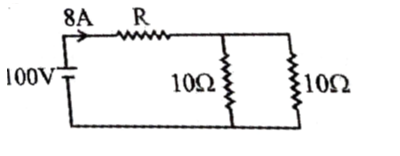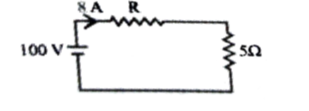Engineering Jobs   »   DFCCIL ELECTRICAL QUIZ,

# DFCCIL’21 EE: Daily Practices Quiz 02-Aug-2021

Each question carries 1 mark.
Negative marking: 1/4 mark
Total Questions: 06
Time: 08 min.

Q1. A star circuit has element of resistance R/2. The equivalent delta elements will be
(a) R/6
(b) 3/2 R
(c) 2R
(d) 4R

Q2. The voltage and current in a circuit are given by:
V=10 sin⁡〖(ωt+π/6)〗
I=10 sin⁡〖(ωt-π/6)〗
The real power consumed is given as……
(a) 25 W
(b) 50 W
(c) 100 W
(d) 86.6 W

Q3. When the plate area of a capacitor increases,
(a) The capacitance increases
(b) The capacitance decreases
(c) The capacitance is unaffected
(d) None of the above

Q4. The value of resistance R in the following circuit is(a) 10 Ω
(b) 5 Ω
(c) 7.5 Ω
(d) 2.5 Ω

Q5. The inductance of a high Q inductor can be measured using a
(a) Schering bridge
(b) Wein bridge
(c) Maxwell bridge
(d) Hay’s bridge

Q6. If the no load voltage of a certain generator is 210 V and the rated voltage is 200 V, then the voltage regulation is
(a)2.5%
(b) 5%
(c) 10 %
(d) 15 %

SOLUTIONS
S1. Ans.(b)
Sol. R/2 → In star connection
∴R_Δ=3*(R/2) → In delta connection

S2. Ans.(a)
Sol. P=V_rms I_rms cos⁡Ө
Where; Ө=Ө_v-Ө_i=π/6-(-π/6)=π/3
∴P=10/√2×10/√2×cos⁡〖(60^0 )=100/4=25 W〗

S3. Ans.(a)
Sol. C=ϵA/d
i.e., C α A
So, when the plate area of a capacitor increases, the capacitance increases.

S4. Ans.(c)
Sol. Both 10 Ω resistance is in parallel.
So, R_eqv=((10×10))/((10+10))=5 Ω
Equivalent circuit:I=V/R_eqv
∴8=100/(R+5)
⇒R=12.5-5=7.5 Ω

S5. Ans.(d)
Sol. The Hay’s bridge is used for determining the self-inductance of the circuit. The bridge is the advanced form of Maxwell’s bridge. The Maxwell’s bridge is only appropriate for measuring the medium quality factor. Hence, for measuring the high-quality factor the Hays bridge is used in the circuit.

S6. Ans.(b)